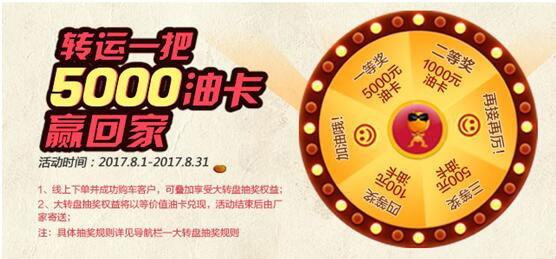## 高温补贴哪家强 幻速工厂直补再省6000

2017年08月08日 12:15 来源：车行天下 超过：13701次关注

今年8月，绝对算得上一个精彩纷呈的月份。一场场燃情大戏，让人们应接不暇。首先是八一建军节90周年大阅兵，场面威武雄壮，气吞山河，让每一个中国人都倍感骄傲；然后是电影《建军大业》和《战狼2》热映，动人心魄的情节画面再次让国人的民族自豪感沸腾起来；再然后，就是北汽幻速8月电商狂欢购车季了。和让人心潮澎湃震撼的场面不一样，北汽幻速让人燃情的是送给消费者的疯狂让利！从8月1日起至8月31日，北汽幻速联合汽车之家和天猫两大电商平台，推出8月狂欢购车季活动，旗下多款车型均有让利优惠， 500元现金抵扣券、1000元油卡、秒杀3000元/5000元金融抵扣券、大转盘抽奖等丰富好礼等你来！ 高温补贴哪家强？首先，北汽幻速在汽车之家平台为广大消费者准备了精彩好礼。凡购买北汽幻速旗下的S2、S3L、S5、H3F、H3五款热销车型均可享受优惠福利,动动手指即可领取现金抵扣券。喜欢北汽幻速S2的消费者，只需99元即可在汽车之家平台上进行预订，预订后成功在线下购车的客户可享1000元油卡，回传发票可得500元油卡，在汽车之家S2论坛发布提车作业贴可再享500油卡，同时，还可以参与店铺大转盘抽奖活动，在线下成功购车后，最高可获得5000元油卡。 高温补贴哪家强？而通过汽车之家预订北汽幻速S3L、S5、H3F、H3四款车型的客户， 99元线上下单，线下购车还可享受抵扣500元车款优惠。除此之外，也可获得店铺大转盘抽奖机会，只要成功购车后，最高奖品为5000元油卡。

整个8月，北汽幻速不仅在汽车之家平台广送福利，在天猫平台同样有好礼狂送不止。活动期间，只需99元即可以天猫线上预订北汽幻速S2 、S3L、S5CVT、H3、H3F四款车型，然后到线下成功购车后，还可享受二重礼遇，分别是购车写好评即获厂商500元抵扣券，和店铺抽奖活动，百分百中奖，最高可得2000元油卡，同时还可重复享受线下其它优惠。

同时，在天猫平台预订北汽幻速S3L、S6、H3F、H3四款热门车型，可参与限时整点秒杀最高5000元分期贷款抵扣券的福利。燃情8月，享燃情好礼！北汽幻速8月电商购车狂欢季现已火热开启！丰富多样的时尚车型，心动实惠的各种让利，只为满足你的燃情汽车梦。别在犹豫了，现在就登陆汽车之家或天猫平台，选购属于你的北汽幻速吧！汽车之家旗舰店链接：

http://store.mall.autohome.com.cn/huansu.html

天猫旗舰店链接：

https://bqhs.tmall.com/index.htm?spm=a1z10.1-b.w5002-15327843013.2.5cdaf1f1KjpZGO

#### 相关文章

0-500 字已有评论 0条 查看评论>>

### 热门标签

﻿
• 快速找车
• 选择品牌
• 选择品牌
• A  奥迪
• A  阿斯顿·马丁
• A  阿尔法·罗密欧
• B  宝沃
• B  布加迪
• B  巴博斯
• B  保时捷
• B  宾利
• B  奔驰
• B  宝马
• B  本田
• B  别克
• B  标致
• B  比亚迪
• B  宝骏
• B  北汽制造
• B  北汽新能源
• B  北汽幻速
• B  北汽威旺
• B  北京汽车
• B  奔腾
• B  北汽绅宝
• C  长安
• C  长安商用
• C  长城
• C  昌河
• D  大众
• D  道奇
• D  DS
• D  东南
• D  东风风神
• D  东风风行
• D  东风小康
• D  东风风度
• D  东风
• F  福特
• F  丰田
• F  菲亚特
• F  法拉利
• F  福田
• F  福迪
• F  福汽启腾
• G  观致
• G  广汽传祺
• G  广汽吉奥
• G  GMC
• H  红旗
• H  汉腾汽车
• H  哈弗
• H  哈飞
• H  海格
• H  海马
• H  华颂
• H  黄海
• H  华泰
• H  恒天
• J  吉利汽车
• J  捷豹
• J  Jeep
• J  江淮
• J  江铃
• J  金杯
• J  九龙
• J  金旅
• K  凯翼
• K  凯迪拉克
• K  克莱斯勒
• K  科尼塞克
• K  卡威
• K  开瑞
• L  路虎
• L  林肯
• L  劳斯莱斯
• L  兰博基尼
• L  雷克萨斯
• L  铃木
• L  雷诺
• L  理念
• L  力帆
• L  莲花汽车
• L  猎豹
• L  路特斯
• L  陆风
• M  马自达
• M  MG
• M  MINI
• M  玛莎拉蒂
• M  摩根
• M  迈凯轮
• N  纳智捷
• O  欧宝
• O  讴歌
• O  欧朗
• Q  奇瑞
• Q  起亚
• Q  启辰
• R  日产
• R  荣威
• R  瑞麒
• S  三菱
• S  斯威汽车
• S  萨博
• S  smart
• S  斯柯达
• S  斯巴鲁
• S  思铭
• S  双龙
• S  上汽大通
• S  双环
• T  特斯拉
• T  腾势
• W  沃尔沃
• W  五菱汽车
• W  五十铃
• W  威兹曼
• W  威麟
• X  现代
• X  雪佛兰
• X  雪铁龙
• X  西雅特
• Y  一汽
• Y  英菲尼迪
• Y  英致
• Y  依维柯
• Y  野马汽车
• Y  永源
• Z  众泰
• Z  中华
• Z  中兴
• Z  知豆
• 选择车系
• 选择车系
• 车型对比
• 选择品牌
• 选择品牌
• A  奥迪
• A  阿斯顿·马丁
• A  阿尔法·罗密欧
• B  宝沃
• B  布加迪
• B  巴博斯
• B  保时捷
• B  宾利
• B  奔驰
• B  宝马
• B  本田
• B  别克
• B  标致
• B  比亚迪
• B  宝骏
• B  北汽制造
• B  北汽新能源
• B  北汽幻速
• B  北汽威旺
• B  北京汽车
• B  奔腾
• B  北汽绅宝
• C  长安
• C  长安商用
• C  长城
• C  昌河
• D  大众
• D  道奇
• D  DS
• D  东南
• D  东风风神
• D  东风风行
• D  东风小康
• D  东风风度
• D  东风
• F  福特
• F  丰田
• F  菲亚特
• F  法拉利
• F  福田
• F  福迪
• F  福汽启腾
• G  观致
• G  广汽传祺
• G  广汽吉奥
• G  GMC
• H  红旗
• H  汉腾汽车
• H  哈弗
• H  哈飞
• H  海格
• H  海马
• H  华颂
• H  黄海
• H  华泰
• H  恒天
• J  吉利汽车
• J  捷豹
• J  Jeep
• J  江淮
• J  江铃
• J  金杯
• J  九龙
• J  金旅
• K  凯翼
• K  凯迪拉克
• K  克莱斯勒
• K  科尼塞克
• K  卡威
• K  开瑞
• L  路虎
• L  林肯
• L  劳斯莱斯
• L  兰博基尼
• L  雷克萨斯
• L  铃木
• L  雷诺
• L  理念
• L  力帆
• L  莲花汽车
• L  猎豹
• L  路特斯
• L  陆风
• M  马自达
• M  MG
• M  MINI
• M  玛莎拉蒂
• M  摩根
• M  迈凯轮
• N  纳智捷
• O  欧宝
• O  讴歌
• O  欧朗
• Q  奇瑞
• Q  起亚
• Q  启辰
• R  日产
• R  荣威
• R  瑞麒
• S  三菱
• S  斯威汽车
• S  萨博
• S  smart
• S  斯柯达
• S  斯巴鲁
• S  思铭
• S  双龙
• S  上汽大通
• S  双环
• T  特斯拉
• T  腾势
• W  沃尔沃
• W  五菱汽车
• W  五十铃
• W  威兹曼
• W  威麟
• X  现代
• X  雪佛兰
• X  雪铁龙
• X  西雅特
• Y  一汽
• Y  英菲尼迪
• Y  英致
• Y  依维柯
• Y  野马汽车
• Y  永源
• Z  众泰
• Z  中华
• Z  中兴
• Z  知豆
• 选择车系
• 选择车系
• 选择车型
• 选择车型
• 意见反馈